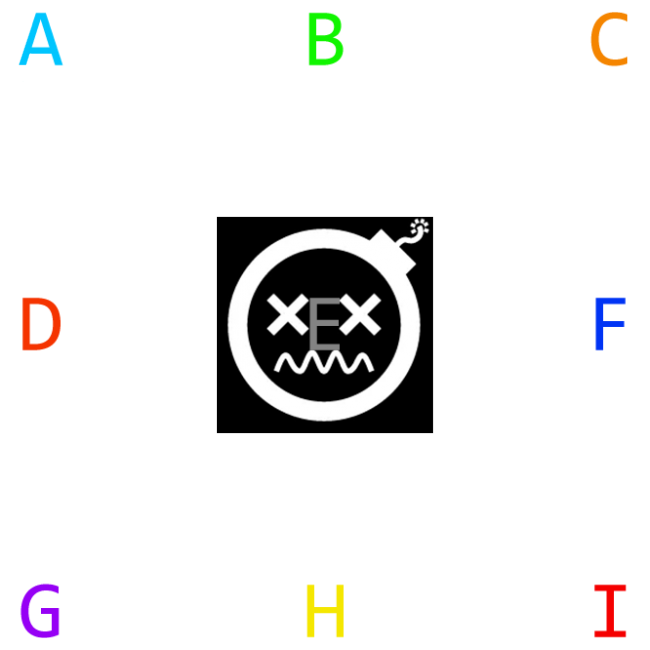#### Howdy, Stranger!

We are about to switch to a new forum software. Until then we have removed the registration on this forum.

#### In this Discussion

edited September 2015

I want to write a shader that takes in a main texture, splits it in 9 equal pieces and outputs only the one in the center, but for each pixel, overlay the corresponding ones from the other 8 pieces added together (actually only one would actually have a color for that pixel, the others would be transparent). How would i go around doing something like that?Thanks!!Tagged:

• edited September 2015 Answer ✓

This should get you started:

``````void setup() {

size(600, 600, P2D);

// Using Processing's texture shader example as base
String[] vertSource = {

"uniform mat4 transform;",
"uniform mat4 texMatrix;",

"attribute vec4 vertex;",
"attribute vec4 color;",
"attribute vec2 texCoord;",

"varying vec4 vertColor;",
"varying vec4 vertTexCoord;",

"void main() {",
"gl_Position = transform * vertex;",

"vertColor = color;",
"vertTexCoord = texMatrix * vec4(texCoord, 1.0, 1.0);",
"}"
};
String[] fragSource = {
"#version 120", // First class arrays require GLSL version 1.20 (or higher)

"#ifdef GL_ES",
"precision mediump float;",
"precision mediump int;",
"#endif",

// Create scale vec2 to map incoming UVs to 1/3 to the "tile" size
"const vec2 scale = vec2(0.3333, 0.3333);",

// Create offset vec2 array to sample from the 8 surrounding "tiles"
"const vec2 offset = vec2[] (",
"vec2(0.0000, 0.0000), vec2(0.3333, 0.0000), vec2(0.6666, 0.0000),",
"vec2(0.0000, 0.3333), vec2(0.6666, 0.3333),",
"vec2(0.0000, 0.6666), vec2(0.3333, 0.6666), vec2(0.6666, 0.6666)",
");",

"uniform sampler2D texture;",

"varying vec4 vertColor;",
"varying vec4 vertTexCoord;",

"void main() {",

// Get the color of the middle "tile"
"vec4 finalColor = texture2D(texture, vertTexCoord.st * scale + vec2(0.3333, 0.3333)) * vertColor;",

// Overlay the 8 surrounding "tiles"
"for(int n = 0; n < offset.length(); ++n) {",
"vec4 tileColor = texture2D(texture, vertTexCoord.st * scale + offset[n]) * vertColor;",
"finalColor.rgb = mix(finalColor.rgb, tileColor.rgb, tileColor.a);",
"}",

// Return the final color
"gl_FragColor = finalColor;",

"}"
};

image(mainTexture, 0, 0, width, height);

}
``````• ;k;

• Sorry, don't know. I never used Unity.